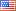# RNG DPS Sheet (BUG)

Eorzea Time

Language: JP EN FR DE
users online
Forum » FFXI » Jobs » Ranger » RNG DPS sheet (BUG)
RNG DPS sheet (BUG)Quetzalcoatl.OrestesOffline
Server: Quetzalcoatl
Game: FFXI
user: Orestes78
Posts: 405
By Quetzalcoatl.Orestes 2018-08-29 10:59:15
Hello,
The "Currently Maintained DPS Sheets" thread is locked, or I would've posted there. The linked DPS sheet for RNG has an error in the formula for calculating relic proc's. (Fully upgraded Yoichi + Annihilator are receiving 5% instead of 13.3%)

Some DPS sheets seem to use 16% relic proc rate, so I've no idea if 13.3% is correct, but it's what the formula above intended.

I have a fixed version here.

If you want to fix it yourself, look at cells F140 and G140 on Data. Here's F140 for reference.
Code
`=IF(OR(LEFT(R.Gear!\$Z5, 11)="Annihilator", LEFT(R.Gear!\$Z5, 12)="Yoichinoyumi"), IF(OR(RIGHT(R.Gear!\$Z5, 2)="95", RIGHT(R.Gear!\$Z5, 2)="99", RIGHT(R.Gear!\$Z5, 2)="119"), 13.3%, 5%), 0)`

The fixed formula for F140.
Code
`=IF(OR(LEFT(R.Gear!\$B5, 11)="Annihilator", LEFT(R.Gear!\$B5, 12)="Yoichinoyumi"), IF(OR(RIGHT(R.Gear!\$B5, 2)="95", RIGHT(R.Gear!\$B5, 2)="99", RIGHT(R.Gear!\$B5, 3)="119", RIGHT(R.Gear!\$B5,7)="119 III"), 13.3%, 5%), 0)`